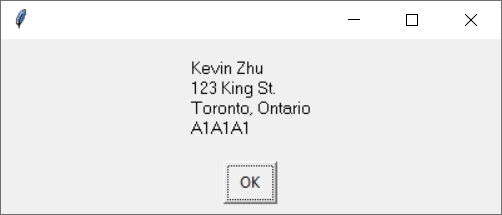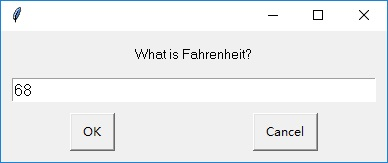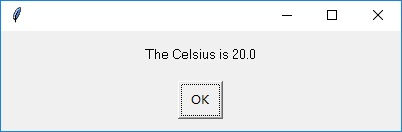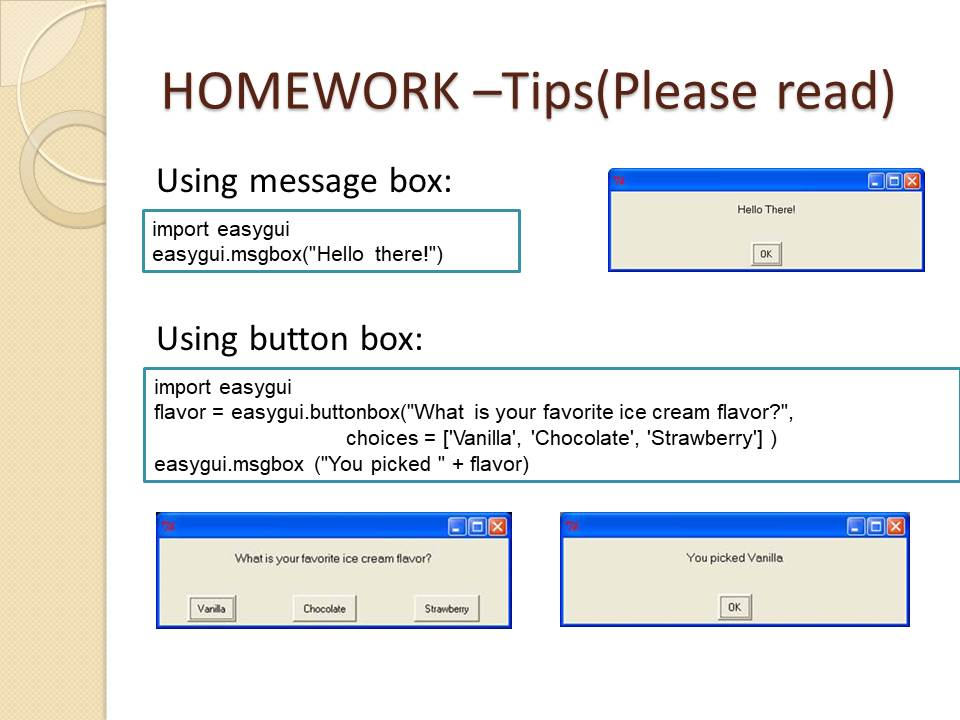TORONTO KIDS COMPUTER CLUB | Summer Camp 2022 Python Homework 13:00 – 07.12.
20831

# Summer Camp 2022 Python Homework 13:00 – 07.12.

## 13 Jul Summer Camp 2022 Python Homework 13:00 – 07.12.

Question 1:
Write a program to help you feed your friends at a party by doing some math about square pizzas. Your program need use input to ask the side length of the square pizza in cm. The area of the pizza should be computed using the formula Area = side length * side length. Then, assuming that each person needs to eat 100 cmof pizza, calculate the number of people it can feed.

Sample Input:
Side length of the square pizza: 17.5

Sample Output:
The pizza can feed 3 people

Explanation and Hint:
If the side length is 17.5, the area will be 306.25 cm2, so 3 is the correct output, because it 3 persons need to eat 300 cm2 in total. You can get this result by using int(), for example:

`people = int(306.25 / 100)`

Question 2:
Write a program to use easygui enterbox that asks for your name, then street, then city, then province, then postal code (all in easygui message boxes). The program should then display a mailing-style full address that looks something like this:```Hint:
Do not forget to import easygui in order to use enterbox and msgbox. You can type the following code to get the input from enterbox:
name = easygui.enterbox('What is your name?')

for example, if we type the following code:

print('Kevin\nZhu')

it will show Kevin and Zhu in two different lines
Kevin
Zhu

```

Question 3:

Use EasyGui to write a program to convert temperatures from Fahrenheit to Celsius. The formula for that is: Celsius = 5 / 9 * (Fahrenheit – 32). Use GUI input and output. You need to create a easygui enterbox to ask Fahrenheit, then use message box to show the Celsius degree. (Hint: the input you get from the enter box is a string, so you need float() to convert it into decimal number.Class review and Hint: